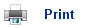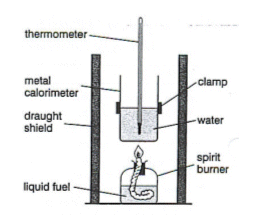# Measuring the enthalpy change of combustion of different fuelsWhen measuring the energy produced from a compound, water is used to measure the amount of heat; this is because of its specific absorption of energy. The experiment above is very inefficient. This is because not all of the energy is absorbed by the water; some is absorbed by the air, while some is absorbed by the container holding the water. Also, the methanol may not have entirely reacted.

## To work out the enthalpy change:

1. Work out the heat given out by the fuel, this can be done by heating up water, as water absorbs a specific amount of energy (4.2J per g).

Mass of water heated x 4.2 x temperature rise

2. Work out the number of moles of fuel that has been burnt. This can be done by working out the difference between the mass of fuel before the water heated, and the mass of fuel afterwards.

Mass
Mr

3. Work out the energy given out by 1 mole of the substance.

Heat given out by the fuel
Number of moles

4. This gives you the J/mol-1, so to get the answer in KJ, divide by 1000.

## Energy given out from Methanol

 Temperature Before: 20oc Temperature After: 20oc Temperature Difference: 20oc

 Initial Mass: 157.57g Final Mass: 155.4g Difference: 2.17g

5. Heat given out by methanol: 200 x 4.2 x 20 = 16800J
6. Number of moles burnt to give heat: 2.17/32 = 0.0678 mol
7. Heat given out by one mole of methanol: 16800J/678= 24787J
8. So Delta HoC (methanol)= -247.8 kJmol-1

Energy given out from Hexane

 Temperature Before: 20oc Temperature After: 30oc Temperature Difference: 10oc

 Initial Mass: 160.12g Final Mass: 159.46g Difference: 0.75g
9. Heat given out by Hexane: 200 x 4.2 x 10 = 8400J
10. Number of moles burnt to give heat: 0.75/86 = 0.0087 mol
11. Heat given out by one mole of Hexane: 8400/0.0087 = 965517J
12. So Delta HoC (methanol)= -965.52 kJmol-1

## Useful books for revision:

Revise AS Chemistry for Salters (Written by experienced examiners and teachers of Salter's chemistry)Revise AS Chemistry for Salters (OCR) (Salters Advanced Chemistry)Home